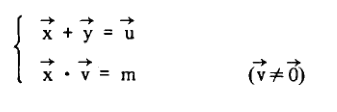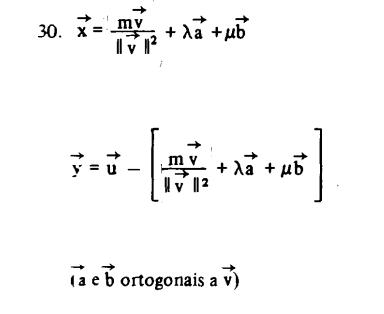# Solve this vector system containing sum and dot product equations

• LCSphysicist
In summary, the conversation discusses a problem involving finding a specific vector in a plane formed by two given vectors. The solution involves writing the unknown vectors in terms of other known vectors and using a unit vector and a constant to generate all possible solutions.f

#### LCSphysicist

Homework Statement
All below
Relevant Equations
All belowSeems to me the answer is a specific vector:

The second forms a plane, while the first X is just a vector. The intersection between the λX that generates the (properties of all vectors that lie in the...) plane (i am not saying X is the director vector!)

How to write this in vector language?

What are you supposed to solve for? The first equation gives ##\vec x## precisely: ##\vec x = \vec u - \vec y##. What more is there to do?

Homework Statement:: All below
Relevant Equations:: All below

View attachment 266376

Seems to me the answer is a specific vector:

The second forms a plane, while the first X is just a vector. The intersection between the λX that generates the (properties of all vectors that lie in the...) plane (i am not saying X is the director vector!)

How to write this in vector language?
You didn't specify whether this is a 2D or 3D problem. You also didn't specify that ##\vec u, ~ \vec v,~ m## are given constants and the unknowns are ##\vec x## and ##\vec y##. Is that correct? Certainly, if it is a 3D problem there can be many solutions. For example if ##\vec v = \langle 0,0,1\rangle## and ##m=0##, so ##\vec x## is perpendicular to ##\vec v## so ##\vec x## can be any vector in the ##xy## plane. Then no matter what ##\vec u## is ##\vec y = \vec u - \vec x## is a solution. So ##\vec u## and ##\vec x ## can be all over the place.

The problem is not i didn't specify, the question is really just it.
Seeing the answer, it write x and y in terms of others vectors a and b.
The answers is right to me, but at same time a little biased, as you both said.The problem is not i didn't specify, the question is really just it.
Seeing the answer, it write x and y in terms of others vectors a and b.
The answers is right to me, but at same time a little biased, as you both said.

View attachment 266391
Unless there was more info provided, I see no way to guess this 'solution' is what was wanted. Indeed, I would propose a better 'solution' is to omit ##\vec b## and define a as a unit vector. ##\frac{m\vec v}{|\vec v|^2}+\lambda\hat a##, where ##\vec v.\hat a=0##, generates all solutions of ##\vec x.\vec v=m##.